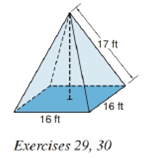Chapter 9.2, Problem 29E### Elementary Geometry for College St...

6th Edition
Daniel C. Alexander + 1 other
ISBN: 9781285195698

#### Solutions

Chapter
Section### Elementary Geometry for College St...

6th Edition
Daniel C. Alexander + 1 other
ISBN: 9781285195698
Textbook Problem
62 views

# A church steeple in the shape of a regular square pyramid needs to be reshingled. The part to be covered corresponds to the lateral area of the square pyramid. If each lateral edge measures 17 f t and each base edge measures 16 f t , how many square feet of shingles need to be replaced?To determine

To find:

The lateral area of the pyramid.

Explanation

Given:

A regular square pyramid as below,

and lateral edge measures 17ft and each base edge measures 16ft.

Properties Used:

A pyramid is made by connecting a base to an apex. The base is flat with straight edges, no curves, hence, a polygon and all other faces are triangles.

A regular pyramid is a pyramid whose base is a regular polygon and whose lateral edges are all congruent.

The lateral area L of a regular pyramid with slant height of length l and perimeter P of the base is given by

L=12lP.

The total surface area T of a pyramid with lateral area L and base area B is given by

T=L+B.

According to the Pythagorean theorem, in a right-angled triangle

hypotenuse2=base2+perpendicular2.

In a regular pyramid, the lengths of the apothem a of the base, the altitude h, and the slant height l satisfy the Pythagorean Theorem; that is, l2=a2+h2.

The perimeter of the square is four times the side.

Calculation:

From the given figure

### Still sussing out bartleby?

Check out a sample textbook solution.

See a sample solution

#### The Solution to Your Study Problems

Bartleby provides explanations to thousands of textbook problems written by our experts, many with advanced degrees!

Get Started

#### In Problems 45-51, solve each system of equations. 51.

Mathematical Applications for the Management, Life, and Social Sciences

#### True or False: If f(x) 0 for x c and f(x) 0 for x c, then c is a point of inflection.

Study Guide for Stewart's Single Variable Calculus: Early Transcendentals, 8th

#### Using for |x| < 1,

Study Guide for Stewart's Multivariable Calculus, 8th